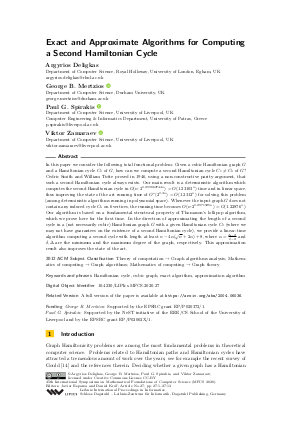Document# Exact and Approximate Algorithms for Computing a Second Hamiltonian Cycle

### Authors Argyrios Deligkas, George B. Mertzios, Paul G. Spirakis, Viktor Zamaraev## File

LIPIcs.MFCS.2020.27.pdf
• Filesize: 0.49 MB
• 13 pages

## Cite As

Argyrios Deligkas, George B. Mertzios, Paul G. Spirakis, and Viktor Zamaraev. Exact and Approximate Algorithms for Computing a Second Hamiltonian Cycle. In 45th International Symposium on Mathematical Foundations of Computer Science (MFCS 2020). Leibniz International Proceedings in Informatics (LIPIcs), Volume 170, pp. 27:1-27:13, Schloss Dagstuhl - Leibniz-Zentrum für Informatik (2020)
https://doi.org/10.4230/LIPIcs.MFCS.2020.27

## Abstract

In this paper we consider the following total functional problem: Given a cubic Hamiltonian graph G and a Hamiltonian cycle C₀ of G, how can we compute a second Hamiltonian cycle C₁ ≠ C₀ of G? Cedric Smith and William Tutte proved in 1946, using a non-constructive parity argument, that such a second Hamiltonian cycle always exists. Our main result is a deterministic algorithm which computes the second Hamiltonian cycle in O(n⋅2^0.299862744n) = O(1.23103ⁿ) time and in linear space, thus improving the state of the art running time of O*(2^0.3n) = O(1.2312ⁿ) for solving this problem (among deterministic algorithms running in polynomial space). Whenever the input graph G does not contain any induced cycle C₆ on 6 vertices, the running time becomes O(n⋅ 2^0.2971925n) = O(1.22876ⁿ). Our algorithm is based on a fundamental structural property of Thomason’s lollipop algorithm, which we prove here for the first time. In the direction of approximating the length of a second cycle in a (not necessarily cubic) Hamiltonian graph G with a given Hamiltonian cycle C₀ (where we may not have guarantees on the existence of a second Hamiltonian cycle), we provide a linear-time algorithm computing a second cycle with length at least n - 4α (√n+2α)+8, where α = (Δ-2)/(δ-2) and δ,Δ are the minimum and the maximum degree of the graph, respectively. This approximation result also improves the state of the art.

## Subject Classification

##### ACM Subject Classification
• Theory of computation → Graph algorithms analysis
• Mathematics of computing → Graph algorithms
• Mathematics of computing → Graph theory
##### Keywords
• Hamiltonian cycle
• cubic graph
• exact algorithm
• approximation algorithm

## Metrics

• Access Statistics
• Total Accesses (updated on a weekly basis)
0

## References

1. Cristina Bazgan, Miklos Santha, and Zsolt Tuza. On the approximation of finding a(nother) hamiltonian cycle in cubic hamiltonian graphs. Journal of Algorithms, 31(1):249-268, 1999.2. Richard Bellman. Dynamic programming treatment of the Travelling Salesman Problem. Journal of the ACM, 9(1):61-63, 1962.3. Andreas Bjorklund. Determinant sums for undirected hamiltonicity. SIAM Journal on Computing, 43(1):280-299, 2014.4. Andreas Björklund and Thore Husfeldt. The parity of directed Hamiltonian cycles. In Profeecings of the 54th Annual IEEE Symposium on Foundations of Computer Science (FOCS), pages 727-735, 2013.5. Andreas Björklund, Thore Husfeldt, Petteri Kaski, and Mikko Koivisto. The traveling salesman problem in bounded degree graphs. ACM Transactions on Algorithms, 8(2):18:1-18:13, 2012.6. Hans L. Bodlaender, Marek Cygan, Stefan Kratsch, and Jesper Nederlof. Deterministic single exponential time algorithms for connectivity problems parameterized by treewidth. Information and Computation, 243:86-111, 2015.7. Kathie Cameron. Thomason’s algorithm for finding a second Hamiltonian circuit through a given edge in a cubic graph is exponential on Krawczyk’s graphs. Discrete Mathematics, 235:69-77, 2001.8. Marek Cygan, Stefan Kratsch, and Jesper Nederlof. Fast hamiltonicity checking via bases of perfect matchings. In Proceedings of the 45th ACM Symposium on Theory of Computing Conference (STOC), pages 301-310, 2013.9. Argyrios Deligkas, George B. Mertzios, Paul G. Spirakis, and Viktor Zamaraev. Exact and approximate algorithms for computing a second hamiltonian cycle. CoRR, 2020. Available online at URL: https://arxiv.org/abs/2004.06036.
10. R.C. Entringer and Henda Swart. Spanning cycles of nearly cubic graphs. Journal of Combinatorial Theory, Series B, 29(3):303-309, 1980.11. David Eppstein. The Traveling Salesman Problem for cubic graphs. Journal of Graph Algorithms and Applications, 11(1):61-81, 2007.12. Herbert Fleischner. Uniqueness of maximal dominating cycles in 3-regular graphs and of Hamiltonian cycles in 4-regular graphs. Journal of Graph Theory, 18(5):449-459, 1994.13. António Girão, Teeradej Kittipassorn, and Bhargav Narayanan. Long cycles in Hamiltonian graphs. Israel Journal of Mathematics, 229(1):269-285, 2019.14. Ronald J. Gould. Recent advances on the Hamiltonian problem: Survey III. Graphs and Combinatorics, 30(1):1-46, 2014.15. Michael Held and Richard M. Karp. A dynamic programming approach to sequencing problems. Journal of the Society for Industrial and Applied Mathematics, 10(1):196-210, 1962.16. Richard M. Karp. Reducibility among combinatorial problems. In Complexity of Computer Computations, pages 85-103. Springer, 1972.17. Adam Krawczyk. The complexity of finding a second Hamiltonian cycle in cubic graphs. Journal of Computer and System Sciences, 58(3):641-647, 2001.18. Maciej Liśkiewicz and Martin R Schuster. A new upper bound for the Traveling Salesman Problem in cubic graphs. Journal of Discrete Algorithms, 27:1-20, 2014.19. Christos H. Papadimitriou. On the complexity of the parity argument and other inefficient proofs of existence. Journal of Computer and system Sciences, 48(3):498-532, 1994.20. Andrew G. Thomason. Hamiltonian cycles and uniquely edge colourable graphs. Advances in Graph Theory, 3:259-268, 1978.21. William T. Tutte. On Hamiltonian circuits. Journal of the London Mathematical Society, 1(2):98-101, 1946.22. Douglas West. Introduction to graph theory. Prentice hall Upper Saddle River, 2 edition, 2001.23. Mingyu Xiao and Hiroshi Nagamochi. An exact algorithm for TSP in degree-3 graphs via circuit procedure and amortization on connectivity structure. Algorithmica, 74(2):713-741, 2016.X

Feedback for Dagstuhl Publishing